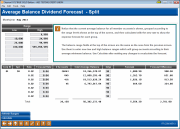# Dividend/Interest Split Rate Forecasting (2)

 Screen ID:  Screen Title:  Panel Number:IEFABD-01 Average Balance Dividend Forecast - Split 200Click here to magnify

This second screen shows the results of the changes in dividend rates, as requested using the variables on the first screen. Notice that the current average balance for all member accounts is shown, grouped according to the range levels shown at the top of the screen, and then calculated with the new rate to show the expense forecast for each group.

The balance range fields at the top of the screen are the same as the ones from the previous screen. Use them to enter new low and high balance ranges which will group accounts according to their average minimum balance. Be sure to use Calculate (F5) after making any changes to recalculate the forecast.

To adjust the forecast for an individual group, enter the new rate into the Forecast Rate field(s) and use F5-Calculate to recalculate the forecast and forecast difference amounts.

## Field Descriptions

 Field Name Description Corp ID The corporation ID selected on the previous screen. DIVAPL The Dividend Application (share product) selected on the previous screen. Rate The current dividend rate for this dividend application. If the product is already set up for a split rate, this will show the rate for each split group. Otherwise, the same rate will be shown for each group. Forecast Rate This column shows the new rate being used to calculate the forecast amount. You may use this column to enter specific rates for individual balance levels. If a change is made, use Calculate (F5)  to recalculate the forecast amounts. # of Accounts The total number of member accounts which fall into each balance range. This is key in measuring the gross member effect of the tier. Sometimes the number of people will be more important than the dollar amounts. Total Avg Balance The total average balance for all member accounts within each group. Average Balance per affected account can be calculated as follows: Total Avg Bal ¸ # of Accts = Average Balance per member Days The number of days of dividends being calculated, as entered on the previous screen. You may use these fields to change the number of days of dividend expense being forecast, and can even use a different number of days for each tier if desired as you experiment. If a change is made, use F5-Calculate to recalculate the forecast amounts. Forecast This column shows the result of the calculation, using the total average balance of all member accounts, at the new rate, for the specified number of days. Average dividend paid per account can be calculated as follows: Forecast ¸ # of Accts = Average dividend per account Forecast Difference This column shows the difference in dividends at the current and forecast rates. Average gain/loss of dividends paid per account can be calculated as follows: Forecast Difference ¸ # of Accts = Average gain/loss per account